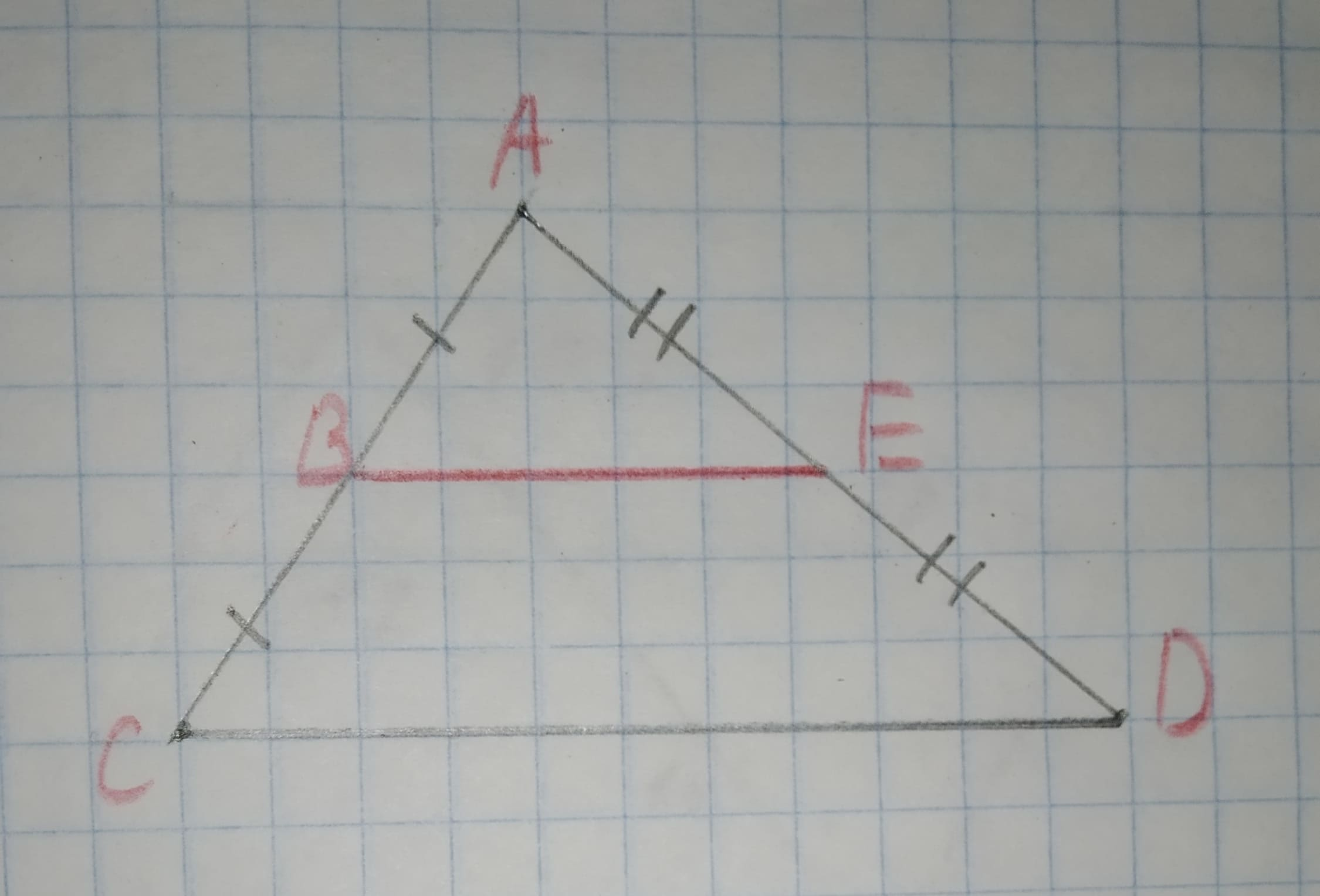# To calculate:The ratio of line AB and BC.Given information:The following diagram is given12210203181.jpgCabiolab 2020-11-01 Answered

To calculate:The ratio of line AB and BC.
Given information:
The following diagram is givenYou can still ask an expert for help

• Questions are typically answered in as fast as 30 minutes

Solve your problem for the price of one coffee

• Math expert for every subject
• Pay only if we can solve ittabuordy

Formula used AAA similarity criterion
Calculation: are similar through AAA similarity.
Therefore,
AB=BC
Therefore, the ratio of AB and BC will be
$\therefore$Ratio of AB and AC is 1:1